# Lecture 26: Redshifted Galaxies

## Redshifted Spiral Nebulae

• 1914 - Vesto Slipher examined the spectra of 15 spiral "nebulae" and found that 11 out of 15 had redshifted spectral lines.
• This meant that an above average number of the "spiral nebulae" were moving away from the Earth.
• Remember: when an object moves away from us, the wavelength of its light is stretched, which causes the spectrum to shift to longer wavelength. (Redshift)
• At this time it was not known that the spiral nebulae were actually separate galaxies.

## Redshifted Galaxies

• In 1923, Edwin Hubble measured the distance to galaxy Andromeda and showed that the distance to Andromeda is bigger than the Milky Way. This showed that the spiral nebulae are really large spiral star systems outside of our galaxy.
• After this Hubble continued to observe galaxies with another astronomer Milton Humason.
• Hubble and Humason observed the spectra of galaxies and observed Cepheid variables in these galaxies.
• By observing the pulsation period of the Cepheid variable stars, they could calculate the distance to the galaxies.
• The also measure the Doppler shifts of spectral lines and interpreted them as velocities of the galaxies. All Doppler shifts were to the red.
• When they plotted velocity versus distance they found strong relation between the two

## Cepheid Variable Stars Used for Distance Measurements

 The Hubble Space Telescope was used to observe the spiral galaxy M100. The inset photos show the Cepheid variable star over a period of a few days. The distance to M100 is 17 Mpc. This is the same method which Hubble used to find the distances to galaxies. One of the major projects that the Hubble Space Telescope was built for was the observation of Cepheid Variable stars in far away galaxies.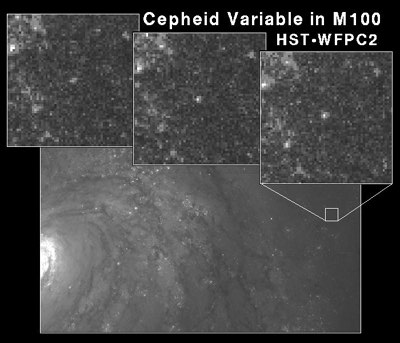## Redshift of Galaxies

• When we look at a galaxy, we expect to see a spectrum similar to the stars inside of it.
• The stars have dark absorption lines corresponding to the elements in their atmospheres.
• If the galaxy has a component of velocity either towards us or away from us, the spectrum of the galaxy will be Doppler shifted due to the motion.
• Define the redshift = z by the equation (&lambda denotes wavelength)

z = (&lambdaobserved - &lambdalab)/&lambdalab

• This is defined so that if the object is moving away from us, the observed wavelength will be longer than expected and z will be positive.
• From the Doppler shift formula, the velocity of the galaxy is
v = c z
• c = speed of light

## Hubble's Law

• Hubble and Humason found a peculiar correlation in the distance and redshift data.
• They found that the larger the distance a galaxy is away from us, the larger the redshift of its spectral lines.
• If the redshift is due to a relative velocity between the galaxy and ourselves, this shows that the further away a galaxy is from us, the faster it moves away from us.
• This is known as Hubbles' Law (although Humason also showed this).
• The mathematical statement of Hubble's law is
v = H0 d
• v = relative velocity of galaxy away from us
• d = distance to the galaxy
• H0 = Hubble's constant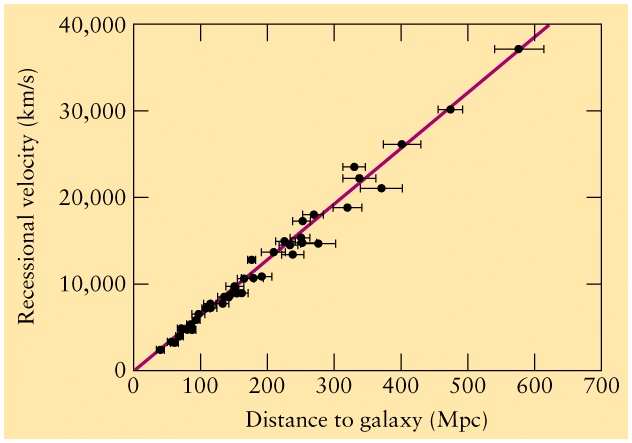### Hubble's Constant and the Hubble Time

• velocity is usually measured in km/s
• distance is usually measured in Mpc
• The slope of the line has units of km/s/Mpc which is a unit of one-over-time.
• Today's best-fit to the slope is H0 = 70 km/s/Mpc
• One over Hubble's constant is a time, called the Hubble time = tH.
• The Hubble time is the time it takes a galaxy to travel from the Milky Way to its present location if it is not accelerating.
• When we look at galaxies outside of the Local Group, we see that all of the galaxies have redshifts that obey Hubble's law.
• The fact that all galaxies seem to be moving away from us suggests that the Universe is expanding uniformly and that the distance between all galaxies is increasing everywhere.
• Hubble time ago, the Universe must look much different than it is know. We conclude that the Universe is evolving
• This is the basis observation which leads to the "Big Bang Model" of the expanding universe which we will study during the last few lectures of this course.

### Warning about large values of z

• Many far away galaxies have redshifts greater than 1.
• This does not mean that the galaxies move faster than light.
• The Doppler shift formula is only valid for small speeds much less than light.
• For speeds which are a large fraction of the speed of light a more complicated relation between z and speed is needed.
• When the correct relativistic formula is used large redshifts are allowed.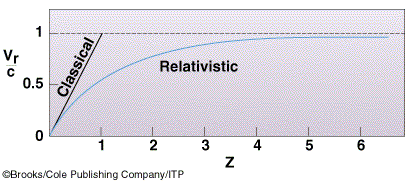## Hubble's Law as a tool for finding distances

• The fact that galaxies obey Hubble's law allows us to use the law to find the distances to galaxies if we have no other way to find the distance.
• The use of Hubble's law allows us to map the distribution of galaxies and clusters of galaxies far away from us.
• Basic idea: It is easy to measure z.
• Distance is then given by:

d = c z/H0
• Since the speed of light and Hubble's constant are known, the distance to the galaxies can be calculated.
• Caveat: if the redshift is very large, a more complicated relativistic formula for d is needed.

## Redshift Surveys of the Universe

• Since distance is proportional to redshift we can make a three dimensional picture of the universe by observing many galaxies and measuring their redshift.
• An important "redshift survey" of about 18,000 galaxies was made from 1985 - 1995 by John Huchra and Margaret Geller.
• In the diagram below, all of the northern hemisphere sky is mapped to the half-circle.
• The white bands with no galaxies are called the "Zone of Avoidance" and is merely the location of the Milky Way's disk which blocks out the light of galaxies.
• Different colours correspond to different ranges of distances away from us.
• This redshift survey shows that at scales of 200 Mpc, galaxies are grouped into large superclusters.
• A supercluster is a large grouping of clusters.
• The Red galaxies near the centre are part of the Virgo Supercluster, also known as the Local Supercluster.
• The dark blue points at the right are the Pisces-Perseus Supercluster
• The light blue (cyan) points at at 15 h are part of the Hercules Supercluster.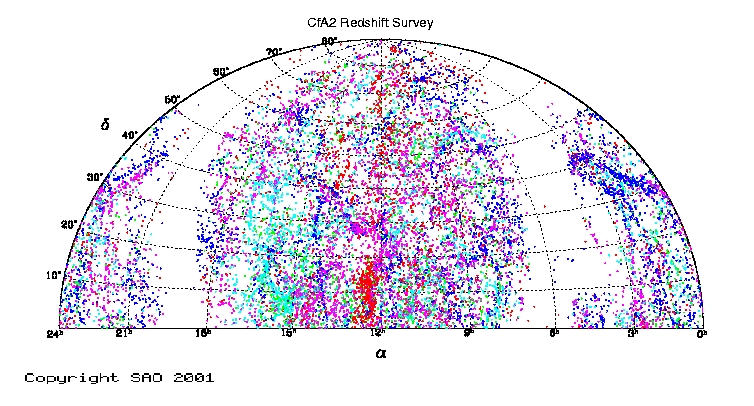## The CfA Redshift Survey

 Colour Max z Max d Red 0.01 43 Mpc Blue 0.02 86 Mpc Magenta 0.03 129 Mpc Cyan 0.04 171 Mpc Green 0.05 214 Mpc

### Modern Redshift Survey: The Cosmic Web

• There are several modern galaxy redshift survey that aim for depth and/or full sky coverage. Prominent example is Sload Digital Sky survey(SDSS)
• It covers the sky in the sequence of wedge-like slices. We can then visualize the distribution of galaxies is to use a 2D slice in the sky where one dimension of position is plotted versus redshift.
• This produces a "wedge" shaped diagram with us at the vertex.
• Distance away from us increases along the straight lines of the wedge. Compute what redshift z=0.25 corresponds to if H0=70 km/s/Mpc
• Another example is 2DF survey (see textbook)
• This diagram shows the distribution of about 930000 galaxies out to a distance of 600 Mpc.
• The Galaxies form filamentary structures woven in the web-like pattern over great distances, with large Voids between them. Hence we speak about the Cosmic Web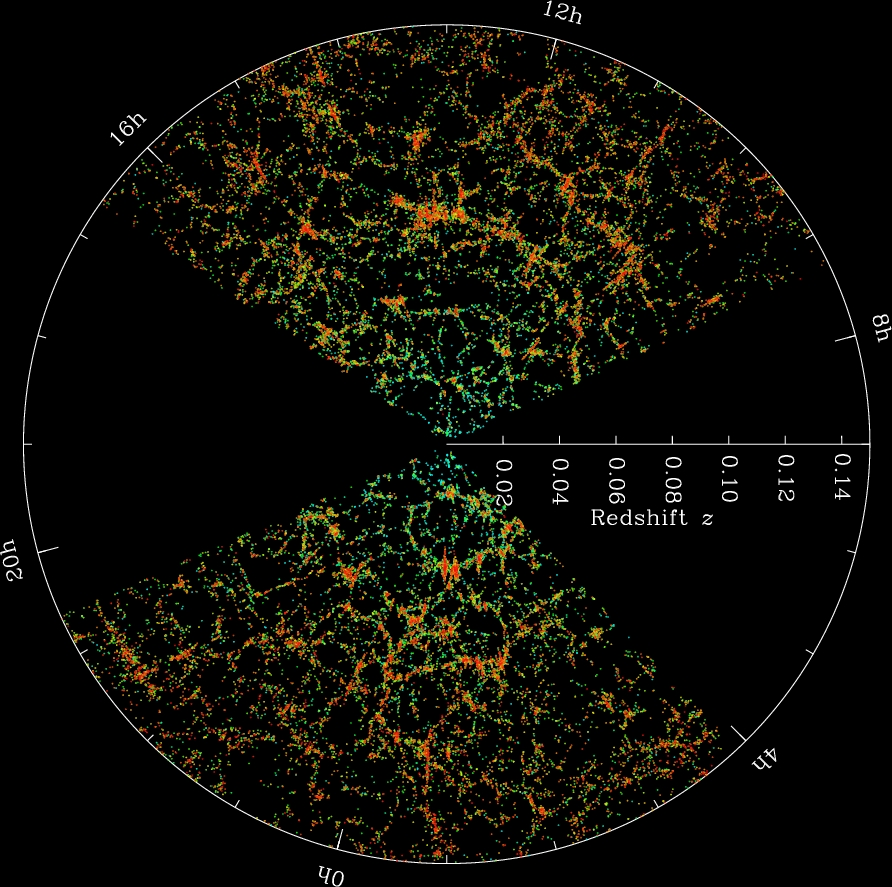## Gravitational Lensing

• When light travels near a massive object, the gravitational field of the object bends the path of the light creating a gravitational lens.
• The gravitational lens can create multiple images or distorted images of a background object emitting the light.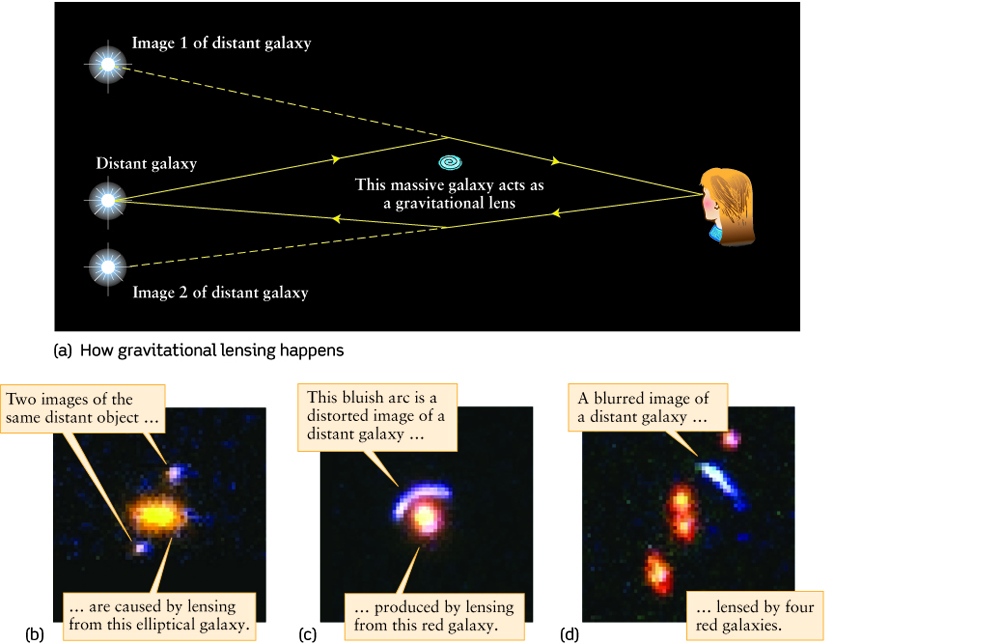### The Einstein Ring

• If there is a perfect alignment between the source, lens and the observer, the image of the source looks like a ring.
• This ring is called an Einstein Ring
• The angular radius of the ring depends on the distance to the lens (d) and the mass of the lens (M):

• If our telescope can't resolve the small angular size of the Einstein Ring, we just see the image's brightness increase. This is called microlensing.
• By examining the image, it is possible to find the mass of the lensing object.
• The bright central region is a nearby galaxy, whose mass acts as a lens for the further away galaxy.
• The image of the far-away galaxy is distorted into a ring shape.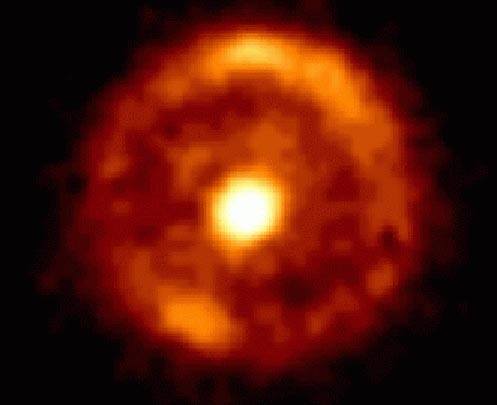## A Galaxy Cluster acting as a Lens

• The Galaxy Cluster Abell 2218 is about 1000 Mpc = Gpc away from us.
• This cluster is acting as a lens.
• Galaxies much further away are lensed by the mass of the cluster into little arc-shapes.
• The lensed arcs are images of galaxies which are 5 - 10 Gpc away.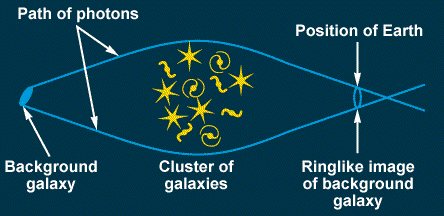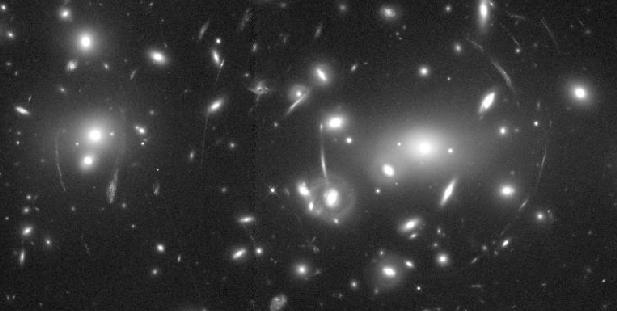### Present record redshift for a galaxy

• The ovals show the location of very faint distorted images of a galaxy with z=7.
• The galaxy is about 13 billion light years away from us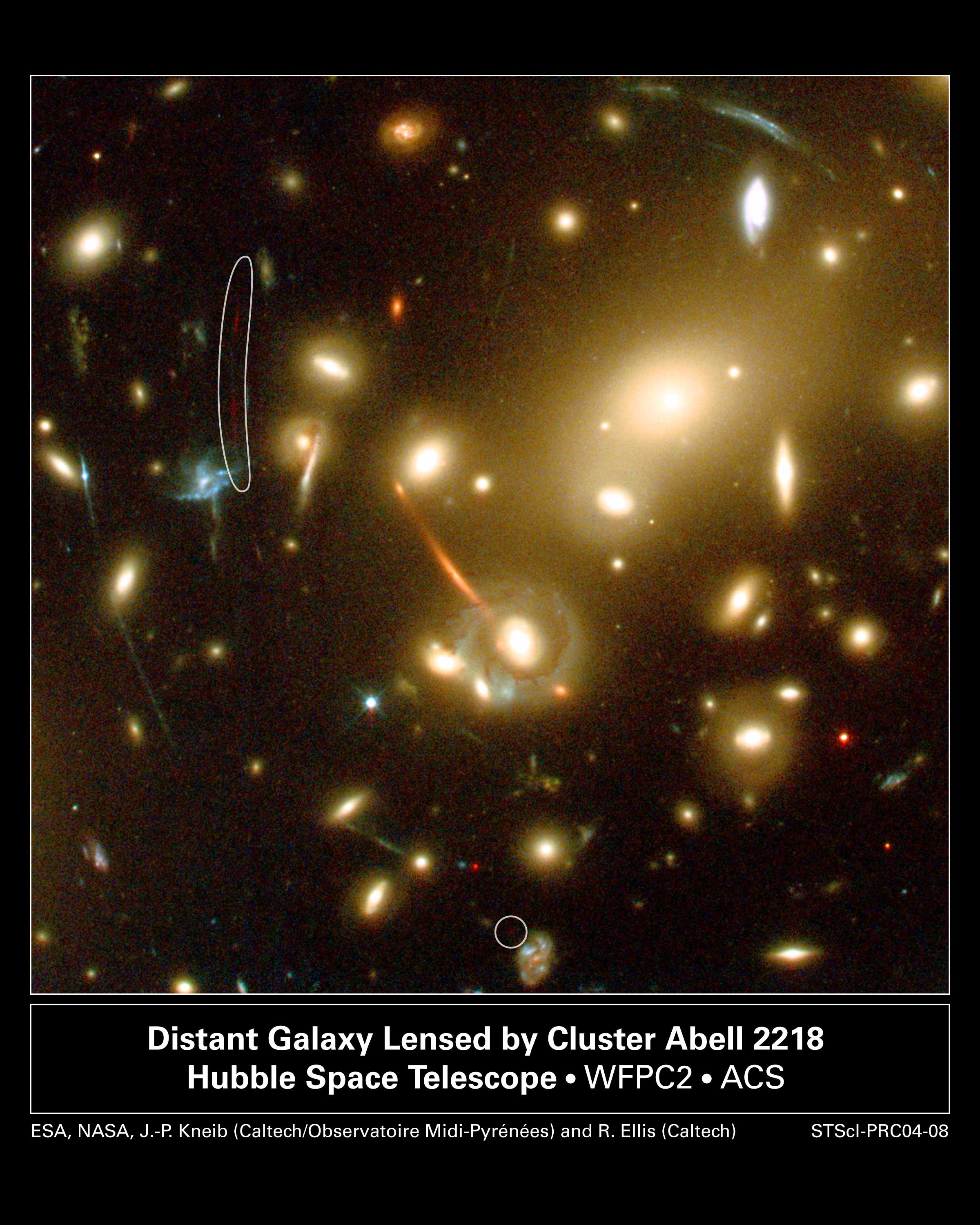### Gravitational Lensing Reveals Dark Matter

• Gravitational lensing of the far away blue galaxy in the bottom left photo is caused by the mass in the galaxy cluster CL0024.
• The galaxies of CL0024 are yellow in the bottom left photo.
• By tracing the paths of light rays we can infer where the mass of the cluster is from the location of the images of the blue galaxy.
• The bottom-right photo shows the inferred location of the cluster's mass in blue.
• The visible galaxies are orange.
• This method infers that 80% of the cluster's mass is not seen in the photo.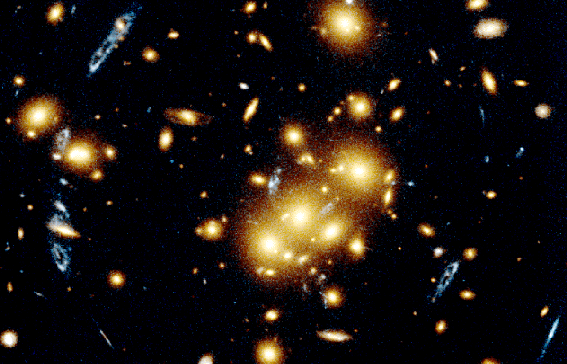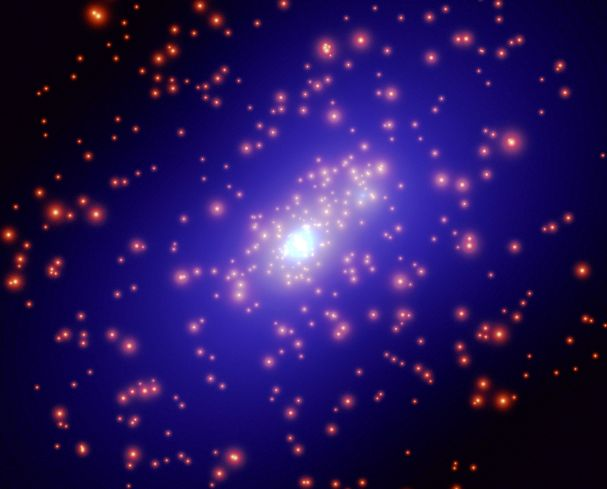### Importance of Lensing

• The observation of lensing is important for two reasons.
• The lensing shows us where invisible mass in a cluster lies.
• The lensing allows us to study distorted images of very young galaxies.
• The general trend of all of these studies is that we only see a small fraction (about 1/10) of the mass of the universe.

Next lecture: Quasars and Active Galaxies